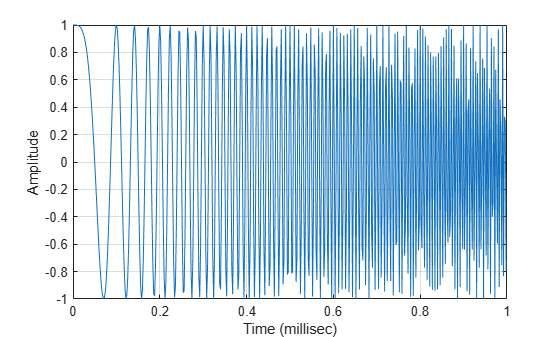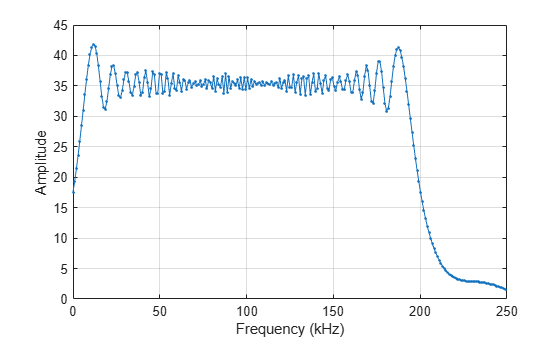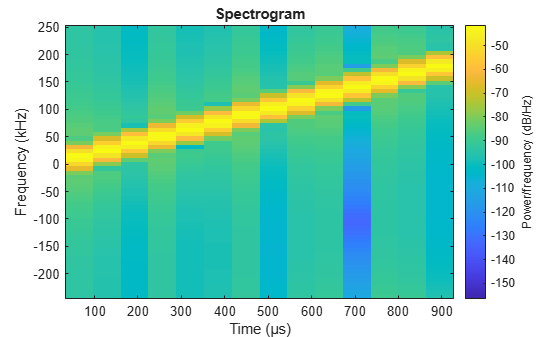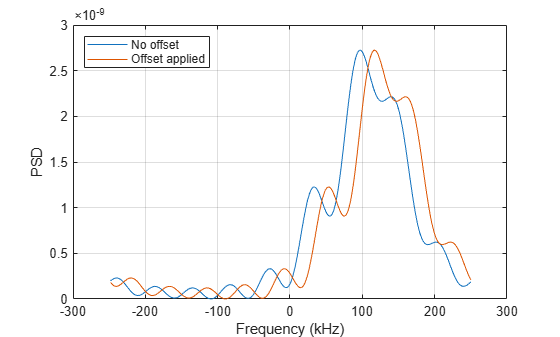# phased.LinearFMWaveform

Linear FM pulse waveform

## Description

The `LinearFMWaveform` object creates a linear FM pulse waveform.

To obtain waveform samples:

1. Define and set up your linear FM waveform. See Construction.

2. Call `step` to generate the linear FM waveform samples according to the properties of `phased.LinearFMWaveform`. The behavior of `step` is specific to each object in the toolbox.

Note

Starting in R2016b, instead of using the `step` method to perform the operation defined by the System object™, you can call the object with arguments, as if it were a function. For example, ```y = step(obj,x)``` and `y = obj(x)` perform equivalent operations. When the only argument to the `step` method is the System object itself, replace `y = step(obj)` by ```y = obj()```.

## Construction

`H = phased.LinearFMWaveform` creates a linear FM pulse waveform System object, `H`. The object generates samples of a linear FM pulse waveform.

`H = phased.LinearFMWaveform(Name,Value)` creates a linear FM pulse waveform object, `H`, with each specified property Name set to the specified Value. You can specify additional name-value pair arguments in any order as (`Name1`,`Value1`,...,`NameN`,`ValueN`).

## Properties

`SampleRate`

Sample rate

Signal sample rate, specified as a positive scalar. Units are Hertz. The ratio of sample rate to pulse repetition frequency (PRF) must be a positive integer — each pulse must contain an integer number of samples.

Default: `1e6`

`DurationSpecification`

Method to set pulse duration

Method to set pulse duration (pulse width), specified as ```'Pulse width'``` or `'Duty cycle'`. This property determines how you set the pulse duration. When you set this property to `'Pulse width'`, then you set the pulse duration directly using the `PulseWidth` property. When you set this property to `'Duty cycle'`, you set the pulse duration from the values of the `PRF` and `DutyCycle` properties. The pulse width is equal to the duty cycle divided by the PRF.

Default: `'Pulse width'`

`PulseWidth`

Pulse width

Specify the length of each pulse (in seconds) as a positive scalar. The value must satisfy `PulseWidth <= 1./PRF`.

Default: `50e-6`

`DutyCycle`

Waveform duty cycle

Waveform duty cycle, specified as a scalar from 0 to 1, exclusive. This property applies when you set the `DurationSpecification` property to ```'Duty cycle'```. The pulse width is the value of the `DutyCycle` property divided by the value of the `PRF` property.

Default: `0.5`

`PRF`

Pulse repetition frequency

Pulse repetition frequency, PRF, specified as a scalar or a row vector. Units are in Hz. The pulse repetition interval, PRI, is the inverse of the pulse repetition frequency, PRF. ThePRF must satisfy these restrictions:

• The product of PRF and PulseWidth must be less than or equal to one. This condition expresses the requirement that the pulse width is less than one pulse repetition interval. For the phase-coded waveform, the pulse width is the product of the chip width and number of chips.

• The ratio of sample rate to any element of `PRF` must be an integer. This condition expresses the requirement that the number of samples in one pulse repetition interval is an integer.

You can select the value of PRF using property settings alone or using property settings in conjunction with the `prfidx` input argument of the `step` method.

• When `PRFSelectionInputPort` is `false`, you set the PRF using properties only. You can

• implement a constant PRF by specifying `PRF` as a positive real-valued scalar.

• implement a staggered PRF by specifying `PRF` as a row vector with positive real-valued entries. Then, each call to the `step` method uses successive elements of this vector for the PRF. If the last element of the vector is reached, the process continues cyclically with the first element of the vector.

• When `PRFSelectionInputPort` is `true`, you can implement a selectable PRF by specifying `PRF` as a row vector with positive real-valued entries. But this time, when you execute the `step` method, select a PRF by passing an argument specifying an index into the PRF vector.

In all cases, the number of output samples is fixed when you set the `OutputFormat` property to `'Samples'`. When you use a varying PRF and set the `OutputFormat` property to `'Pulses'`, the number of samples can vary.

Default: `10e3`

`PRFSelectionInputPort`

Enable PRF selection input

Enable the PRF selection input, specified as `true` or `false`. When you set this property to `false`, the step method uses the values set in the `PRF` property. When you set this property to `true`, you pass an index argument into the `step` method to select a value from the PRF vector.

Default: `false`

`SweepBandwidth`

FM sweep bandwidth

Specify the bandwidth of the linear FM sweeping (in hertz) as a positive scalar. The default value corresponds to 100 kHz.

Default: `1e5`

`SweepDirection`

FM sweep direction

Specify the direction of the linear FM sweep as one of `'Up'` or `'Down'`.

Default: `'Up'`

`SweepInterval`

Location of FM sweep interval

If you set this property value to `'Positive'`, the waveform sweeps in the interval between 0 and B, where B is the `SweepBandwidth` property value. If you set this property value to `'Symmetric'`, the waveform sweeps in the interval between –B/2 and B/2.

Default: `'Positive'`

`Envelope`

Envelope function

Specify the envelope function as one of `'Rectangular'` or `'Gaussian`'.

Default: `'Rectangular'`

`FrequencyOffsetSource`

Source of frequency offset

Source of frequency offset for the waveform, specified as `'Property'` or `'Input port'`.

• When you set this property to `'Property'`, the offset is determined by the value of the `FrequencyOffset` property.

• When you set this property to `'Input port'`, the `FrequencyOffset` is determined by the `freqoffset` input argument.

Default: `'Property'`

`FrequencyOffset`

Frequency offset

Frequency offset in Hz, specified as a scalar.

#### Dependencies

This property applies when you set the `FrequencyOffsetSource` property to `'Input port'`.

Default: `0` Hz

`OutputFormat`

Output signal format

Specify the format of the output signal as `'Pulses'` or `'Samples'`. When you set the `OutputFormat` property to `'Pulses'`, the output of the `step` method takes the form of multiple pulses specified by the value of the `NumPulses` property. The number of samples per pulse can vary if you change the pulse repetition frequency during the simulation.

When you set the `OutputFormat` property to `'Samples'`, the output of the `step` method is in the form of multiple samples. In this case, the number of output signal samples is the value of the `NumSamples` property and is fixed.

Default: `'Pulses'`

`NumSamples`

Number of samples in output

Specify the number of samples in the output of the `step` method as a positive integer. This property applies only when you set the `OutputFormat` property to `'Samples'`.

Default: `100`

`NumPulses`

Number of pulses in output

Specify the number of pulses in the output of the `step` method as a positive integer. This property applies only when you set the `OutputFormat` property to `'Pulses'`.

Default: `1`

`PRFOutputPort`

Set this property to `true` to output the PRF for the current pulse using a `step` method argument.

#### Dependencies

This property can be used only when the `OutputFormat` property is set to `'Pulses'`.

Default: `false`

`CoefficientsOutputPort`

Enable matched filter coefficients output port

Enable the matched filter coefficients output port, specified as `false` or `true`. When you set this property to `false`, the object does not provide the matched filter coefficients used during the simulation as an output. When you set this property to `true`, the object provides the matched filter coefficients used during the simulation as an output.

Default: `false`

## Methods

 bandwidth Bandwidth of linear FM waveform getMatchedFilter Matched filter coefficients for waveform getStretchProcessor Create stretch processor for waveform plot Plot linear FM pulse waveform reset Reset states of the linear FM waveform object step Samples of linear FM pulse waveform
Common to All System Objects
`release`

Allow System object property value changes

## Examples

collapse all

Create and plot an upsweep linear FM pulse waveform. The sample rate is 500 kHz, the sweep bandwidth is 200 kHz and the pulse width is 1 millisecond (equal to the pulse repetition interval).

```fs = 500e3; sLFM = phased.LinearFMWaveform('SampleRate',fs,... 'SweepBandwidth',200e3,... 'PulseWidth',1e-3,'PRF',1e3);```

Obtain and then plot the real part of the LFM waveform.

```lfmwav = step(sLFM); nsamp = size(lfmwav,1); t = [0:(nsamp-1)]/fs; plot(t*1000,real(lfmwav)) xlabel('Time (millisec)') ylabel('Amplitude') grid```Plot the Fourier transform of the complex signal.

```nfft = 2^nextpow2(nsamp); Z = fft(lfmwav,nfft); fr = [0:(nfft/2-1)]/nfft*fs; plot(fr/1000,abs(Z(1:nfft/2)),'.-') xlabel('Frequency (kHz)') ylabel('Amplitude') grid```Plot a spectrogram of the function with window size of 64 samples and 50% overlap.

```nfft1 = 64; nov = floor(0.5*nfft1); spectrogram(lfmwav,hamming(nfft1),nov,nfft1,fs,'centered','yaxis')```This plot shows the increasing frequency of the signal.

Apply a frequency offset to an upsweep linear FM (LFM) pulse waveform. Plot the frequency spectrum of the waveform with and without applying a frequency offset.

Create an LFM waveform object, which is configured to set the frequency offset from an input when the object is executed.

```fs = 500e3; sLFM = phased.LinearFMWaveform('SampleRate',fs,'SweepBandwidth',200e3, ... 'PulseWidth',2e-5,'PRF',1e3,'FrequencyOffsetSource','Input port');```

Execute the object two times. First set the frequency offset to 0 Hz and then to 2e4 Hz.

```lfmwav = sLFM(0); lfmwav_foffset = sLFM(2e4);```

Plot the frequency spectrum of the complex signals. The frequency offset signal is shifted to the right.

```[Pxx,f] = pwelch(lfmwav,[],[],[],fs,'centered'); [Pxx_offset,foffset] = pwelch(lfmwav_foffset,[],[],[],fs,'centered'); plot(f/1000,Pxx,foffset/1000,Pxx_offset) ylabel('PSD'); xlabel('Frequency (kHz)'); legend({'No offset','Offset applied'},'Location','northwest'); grid on;```## References

 Levanon, N. and E. Mozeson. Radar Signals. Hoboken, NJ: John Wiley & Sons, 2004.

 Richards, M. A. Fundamentals of Radar Signal Processing. New York: McGraw-Hill, 2005.

## Version History

Introduced in R2011a